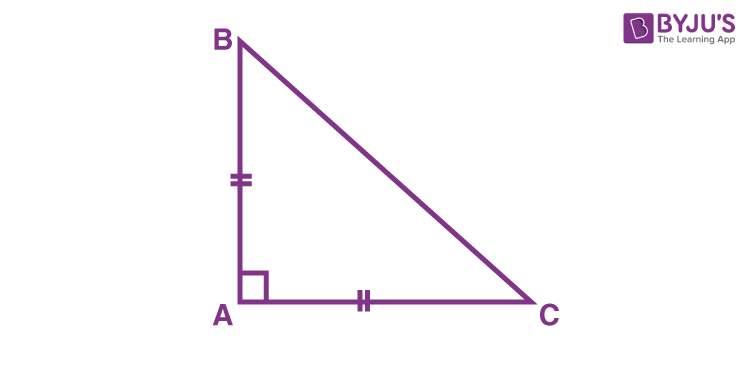# ABC is a right-angled triangle in which ∠A=90° and AB=AC. Find ∠B and ∠C.AB=AC

Therefore, ΔABC is a right-angled isosceles

∠B=∠C (angles opposite to equal sides are equal)

∠A+∠B+∠C=180° (angle-sum property of a triangle)

Substituting ∠B = ∠C, ∠A = 90°

90°+2∠B=180°

2∠B=180°-90°=90°

∠B=45°

∠C=∠B=45°

Image pending(1)(1)